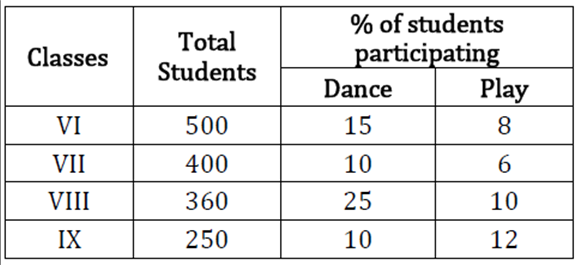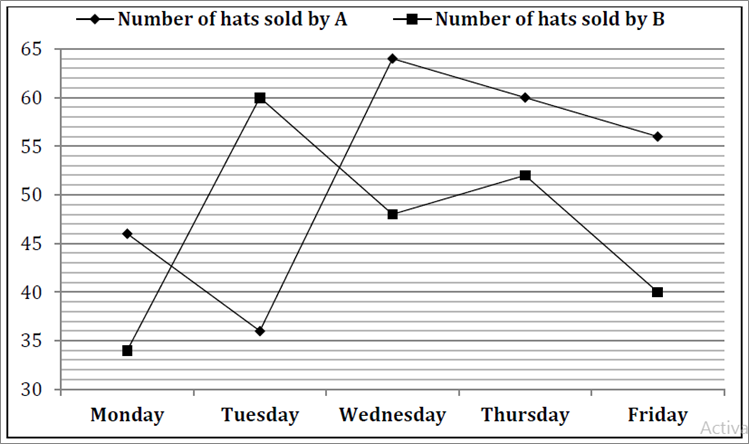# SBI Clerk Numerical Ability Online Test Questions Test 8

1.
Directions(Q.1 to Q.6 ):

Given below, the table which shows the total students in 4 different class of a school and percentage of students participating in Dance and play from these 4 classes.What is the ratio between students participating in Dance from Class VII and IX together to the students participating in Play from class VI and VIII together

a

43 : 53

b

65 : 76

c

44 : 57

d

63 : 71

e

62 : 77

2.

What is the average of students who participating in Play from all class.

a

$32frac{1}{2}$

b

$34frac{1}{2}$

c

$27frac{1}{2}$

d

$35frac{1}{2}$

e

$30frac{1}{2}$

3.

Students who are participating in dance from class VII are what percent more or less than students who are participating is play from class IX.

a

$12frac{2}{7}{ m{\% }}$

b

$14frac{2}{7}{ m{\% }}$

c

$33frac{1}{3}{ m{\% }}$

d

$16frac{2}{3}{ m{\% }}$

e

$66frac{2}{3}{ m{\% }}$

4.

What is the sum of students who does not participate in dance and play from class VI and IX together.

a

720

b

480

c

620

d

580

e

560

5.

If 20% of students who participate in dance from class VI also participate in play then find the ratio of students from class VI who participated only in Dance to students participated only in play.

a

12 : 5

b

16 : 25

c

19 : 20

d

20 : 19

e

15 : 11

6.

Students participating in Dance from class VII is what percent of students participating in play from class IX.

a

$33frac{1}{3}{ m{\% }}$

b

$120frac{2}{7}{ m{\% }}$

c

$114frac{2}{7}{ m{\% }}$

d

$133frac{1}{3}{ m{\% }}$

e

$116frac{2}{3}{ m{\% }}$

7.
Directions(Q.7 to Q.11 ):

What should come in place of question mark (?) in the following number series?

3, 5, 15, 45, 113, ?

a

190

b

234

c

293

d

243

e

208

8.

17, 98, 26, 89, 35, ?

a

78

b

79

c

80

d

81

e

82

9.

3240, 540, 108, 27, ?, 4.5

a

12

b

7

c

9

d

8

e

6

10.

7, 4.5, 5.5, 12, 49, ?

a

393

b

378

c

197

d

148

e

246

11.

2, 17, 89, 359, 1079, ?

a

2134

b

1081

c

2195

d

2159

e

1945

12.
Directions(Q.17 to Q.23 ):

Find out of the approximate value of ? in the following questions

$left( {sqrt {80.997} - sqrt {25.001} } ight) imes (sqrt {120.90} + sqrt {16.02)} = ?$

a

50

b

60

c

75

d

70

e

55

13.

55.01 – 345.02 ÷ 22.99 = 2×?

a

20

b

25

c

22

d

15

e

18

14.

$sqrt {3099.985 div 62.001 + 14.001} = ?$

a

4

b

8

c

6

d

9

e

5

15.

(111.99×5)÷14.02=11.002+ ?

a

34

b

19

c

39

d

29

e

38

16.

24.97% of 84.01 ÷ 6.995 = ?

a

3

b

8

c

5

d

7

e

6

17.

$left( {184.002 - frac{{29}}{5}} ight) imes 29.99 = ?$

a

4950

b

4820

c

5550

d

5346

e

5260

18.

Sum of present age of A, B, C and D is 76 years. After 7 years ratio of their ages is 7 : 6 : 5 : 8. What is C’s present age.

a

14

b

12

c

13

d

8

e

10

19.
Directions(Q.24 to Q.34 ):

Sum of length of two trains A and B is 660. Ratio of speed of A and B is 5 : 8. Ratio between time to cross and electric pole by A and B is 4 : 3. Find the difference in the length of two trains.

a

50

b

60

c

80

d

75

e

90

20.

A mixture of milk and water in a jar contains 28 L milk and 8 L water. X L milk and X L water is mixed in the mixture. If 40% of the new mixture is 20 L, then find the value of X.

a

7 L

b

8 L

c

6 L

d

5 L

e

9 L

21.

A alone can do a work in 24 days. Time taken by A in completing 1/3 of work is equal to the time taken by B in completing 1/2 of the work. In what time A and B together will complete the work.

a

9 days

b

10 days

c

12 days

d

9 $frac{3}{5}$ days

e

8 days

22.

Marked priced of A is 1600 Rs more than its cost price. When discount on A is 500 there is a profit of 25% obtained. At what price A should be sold to obtain 30% profit.

a

4800

b

5600

c

5400

d

5200

e

5720

23.

A three digits number X, which tenth’s place digit is 3. If the unit digit and hundred place digit of number x interchanged thus the number formed is 396 more than the previous one. If the sum of unit digit and hundred digit is 14, then what is the number?

a

480

b

539

c

593

d

935

e

None of these

24.

S1 is a series of 4 consecutive even numbers. If the sum of reciprocal of first two numbers of S1 is 11/60, then what is the reciprocal of third highest number of S1

a

$frac{2}{{13}}$

b

$frac{1}{{12}}$

c

$frac{2}{{17}}$

d

$frac{1}{{13}}$

e

None of these

25.

A, B and C invested in a business in the ratio 6 : 8 . B invested for a period whose numerical value is 112.5% of his investement and A and C invested for one year. If profit of B at the end of year is 16750, then what is the share of profit of C.

a

20225

b

22125

c

25225

d

25125

e

23125

26.

Ratio of cost price to selling price of an article is 5 : 6. If 20% discount is offered on marked price of article then marked price is what percent more than cost price?

a

$frac{{100}}{3}{ m{;\% }}$

b

50%

c

40%

d

$frac{{200}}{3}{ m{\% }}$

e

60%

27.

Ramesh has 20% savings with him from his monthly salary. If expenditure on clothing is 25% of overall expenditure and his total expenditure except clothing is 3600 then find his saving.

a

1000

b

1500

c

1600

d

1200

e

900

28.
Directions(Q.35 to Q.40 ):

A bar graph is given below which shows hat sold by seller A and seller B on five days.Total number of hats sold by A and B together on Wednesday is how much percentage more than the number of hats sold by A and B together on Tuesday:

a

$15frac{2}{3}{ m{\% }}$

b

$8frac{1}{3}{ m{\% }}$

c

$16frac{2}{5}{ m{\% }}$

d

$16frac{2}{3}{ m{\% }}$

e

$21frac{3}{7}{ m{\% }}$

29.

If number of hats sold on Friday by A is increases by 14${ m{;}}frac{2}{7}$ % , then what will be the average no. of hats sold on Monday, Wednesday and Friday by A?

a

85

b

58

c

56

d

82

e

52

30.

Find the number of hats sold on Saturday by A and B together, if number of hats sold on Saturday is

7$frac{1}{7}$ % more than the hats sold on Thursday by A and B together?

a

110

b

114

c

116

d

118

e

120

31.

What is the difference between the number of hats sold on Monday and Wednesday by B to the number of hats sold on Friday by both A & B together.

a

9

b

12

c

14

d

21

e

24

32.

A sold 80% defective hats on Thursday and B sold 75% defective hats on same day. Then find the number of hats sold by A and B on Thursday that are not defected?

a

25

b

20

c

18

d

32

e

40

33.

Find the ratio of number between hats sold by A on Tuesday & Friday together to number of hats sold by B on same days.

a

25 : 23

b

23 : 25

c

21 : 25

d

25 : 21

e

18 : 17

Rate This:
NaN / 5 - 1 votes

### SBI Clerk Numerical Ability Online Test Questions Test 8

» Your comments will be displayed only after manual approval.